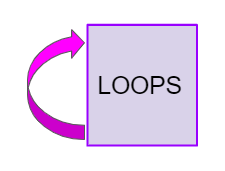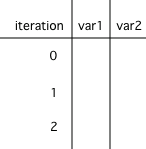# While Loops¶When you play a song, you can set it to loop, which means that when it reaches the end it starts over at the beginning. A loop in programming, also called iteration or repetition, is a way to repeat one or more statements. If you didn’t have loops to allow you to repeat code, your programs would get very long very quickly! Using a sequence of code, selection (ifs), and repetition (loops), the control structures in programming, you can construct an algorithm to solve almost any programming problem!

A `while` loop executes the body of the loop as long as (or while) a Boolean condition is true. When the condition is false, we exit the loop and continue with the statements that are after the body of the `while` loop. If the condition is false the first time you check it, the body of the loop will not execute.

Notice the `while` statement looks a lot like an `if` statement, but it runs more than once. The curly brackets { } are optional when there is just 1 statement following the condition, but required if there are more than 1 statement in the loop. Always using curly brackets is a good practice to follow.

```// if statements just run once if the condition is true
if (condition)
{
statements;
}
// while statements are repeated while the condition is true
while (condition)
{
statements;
}
```

Here’s what the flow of control looks like in a Java while loop. Notice that while the condition is true, the loop body is repeated.

The loop condition usually involves a loop control variable that controls when to stop the loop. The simplest loops are counter-controlled loops like below, where the loop variable is a counter that controls how many times to repeat the loop. There are 3 steps to writing a loop using this loop control variable as seen below in a loop that counts from 1 to 10.

Note

Remember these 3 steps to writing a loop:

1. Initialize the loop variable (before the while loop)

2. Test the loop variable (in the loop header)

3. Change the loop variable (in the while loop body at the end)

The `LoopTest1` program contains a while loop that counts from 1 to 5 that demonstrates the 3 steps of writing a loop. Use the debugger to step through the execution. Can you change it to count from 2 to 10?

Java doesn’t require your code to be correctly indented (code moved to the right a few spaces) to make it clear what statements are part of the body of the loop, but it is standard practice to do so.Check your understanding

## Tracing Loops¶

A really important skill to develop is the ability to trace the values of variables and how they change during each iteration of a loop.

You can create a tracing table that keeps track of the variable values each time through the loop as shown below.Figure 5: A trace table showing the values of all of the variables each time through the loop. Iteration 0 means before the loop.¶

Watch the following video for a tracing demo. When you are tracing through code, pretend to be the computer running the code line by line, repeating the code in the loop, and keeping track of the variable values and output.Check your understanding

Step through the code above with the visualizer.

## Common Errors with Loops¶

One common error with loops is infinite loops. An infinite loop is one that never stops (the condition is always true).

```// an infinite loop
while (true)
{
System.out.println("This is a loop that never ends");
}
```

The infinite loop above is pretty obvious. But, most infinite loops are accidental. They usually occur because you forget to change the loop variable in the loop (step 3 of a loop).

Another common error with loops is an off-by-one error where the loop runs one too many or one too few times. This is usually a problem with step 2 the test condition and using the incorrect relational operator < or <=.

The while loop in `LoopTest2` should print out the numbers 1 to 8, but it has 2 errors that cause an infinite loop and an off-by-one error. Can you fix the errors? If you run an infinite loop, you may need to refresh the page to stop it (so make sure all active code windows on the page have been saved and click on Load History after refreshing).

## Summary¶

• Iteration statements (loops) change the flow of control by repeating a set of statements zero or more times until a condition is met.

• Loops often have a loop control variable that is used in the boolean condition of the loop. Remember the 3 steps of writing a loop:

• Initialize the loop variable

• Test the loop variable

• Change the loop variable

• In loops, the Boolean expression is evaluated before each iteration of the loop body, including the first. When the expression evaluates to true, the loop body is executed. This continues until the expression evaluates to false which signals to exit the loop. If the Boolean expression evaluates to false initially, the loop body is not executed at all.

• A loop is an infinite loop when the Boolean expression always evaluates to true so that the loop never ends.

• Off by one errors occur when the iteration statement loops one time too many or one time too few.

• If the Boolean expression evaluates to false initially, the loop body is not executed at all.

• There are standard algorithms to compute a sum or average.